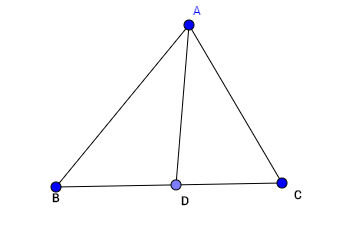# Eccentric givens!

Geometry Level 4Given that in triangle $ABC$ above, $AD$ bisects $\angle CAB$. Find $AD$, given that $(AB) \cdot (AC) = 10$ and $(BD) \cdot(DC) = 6$.

×